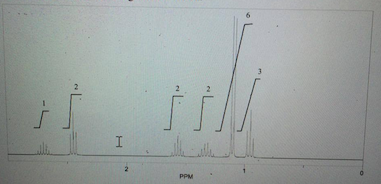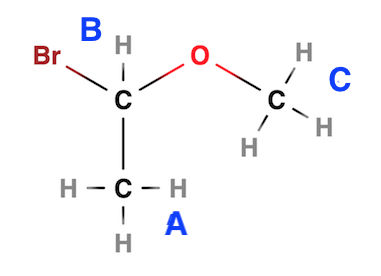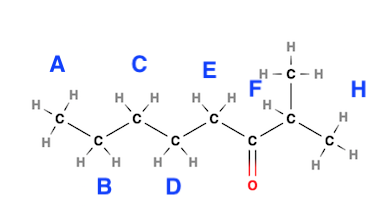# What is the structure of the compound in the following H - NMR spectrum with the molecular...

## Question:

What is the structure of the compound in the following H - NMR spectrum with the molecular formula {eq}C_8H_{16}O {/eq}? Relative integration is shown.## NMR

The hydrogens split based on hydrogens on adjacent atoms. The magnitude of the peaks (area under the peak) tells us how many hydrogens are being split. The number of adjacent hydrogens determines the splitting. Zero adjacent hydrogens gives a singlet, one adjacent hydrogen gives a doublet, two adjacent hydrogens gives a triplet, three adjacent hydrogens gives a quartet, and so on and so forth. The shift (or delta or peak location) is based on the number and type of electronegative group(s) present such as carbonyls, alcohols, esters, halogens, etc. Also, the shifts occur when the hydrogen is bonded to non-carbon atoms such as N or O.

For H or proton NMR, spin Multiplicity plays a role in determining the number of neighboring protons using the n+1 rule which gives the number of peaks present for a given signal. The "n" is the number of neighboring protons.On the example molecule, the hydrogens in group A and B are considered neighbors or adjacent to one another when it comes to peak splitting. In contrast, the hydrogens in group C are not adjacent or neighboring an other hydrogens. {eq}H_B {/eq} has 3 neighboring protons; thus, the it will have a signal with four peaks (quartet), or in mathematical terms 3+1=4. {eq}H_A {/eq} has 1 neighboring protons; thus, the it will have a signal with two peaks (doublet) or in mathematical terms 1+1=2. {eq}H_C {/eq} has 0 neighboring protons; thus, the it will have a signal with two peaks (singlet) or in mathematical terms 0+1=1.The degrees of unsaturation adds up to 1 and non of the HNMR peaks are on alkenes, and there is an O present; thus, there is a carbonyl.

• The...

Become a Study.com member to unlock this answer! Create your account